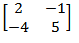Related Articles
GATE | GATE-CS-2005 | Question 49
• Difficulty Level : Medium
• Last Updated : 12 Sep, 2014

What are the eigenvalues of the following 2 × 2 matrix?(A) -1 and 1
(B) 1 and 6
(C) 2 and 5
(D) 4 and -1

Answer: (B)

Explanation: The eigenvalues of A are precisely the real numbers λ that satisfy the equation det(A – &lambda I) = 0.

Let us find determinant value of A – &lambda I;

```2-λ     -1
-4       5-λ
```

We get the equation as λ2 -7 λ + 6 = 0 which gives us eigenvalues as 6 and 1.

Quiz of this QuestionMy Personal Notes arrow_drop_up